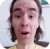FreeKB - Perl Determine if a variable is empty or null
Perl - Determine if a variable is empty or nullLet's say you want to determine if the \$foo variable is empty or contains any value. In this example, the \$foo variable has not been defined. Notice there is more than way comparison that can be done to determine if \$foo is empty or contains a value.

``````#!/usr/bin/perl

use strict;
use warnings;

if (\$foo)       { print "the \\$foo variable contains a value of \$foo\n"; }
if (\$foo ne "") { print "the \\$foo variable contains a value of \$foo\n"; }

if (not \$foo)   { print "the \\$foo variable is empty (contains no value)\n"; }
if (\$foo eq "") { print "the \\$foo variable is empty (contains no value)\n"; }``````

Running this script will return the following.

``````Global symbol "\$foo" requires explicit package name at example.pl line 6.
Execution of example.pl aborted due to comilation errors.``````

This is expected since use strict and use warnings are being used. Let's create the \$foo variable.

``````my \$foo;
``````

Now the script returns the following since we did not give \$foo any value.

``the \$foo variable is empty (contains no value)``

Let's define \$foo with an empty value.

``my \$foo = "";``

Now the script returns the following since we did not give \$foo any value.

``the \$foo variable is empty (contains no value)``

Let's give \$foo a value of 0;

``my \$foo = 0;``

The script still return the following, which is kind of interesting. In this way, we can see that 0 is the same as undefined or empty.

``the \$foo variable is empty (contains no value)``

Finally, lets give foo any value.

``my \$foo = "bar";``

Now the script identifies that the \$foo variable has a value.

``the \$foo variable contains a value of bar``

We will never share your name or email with anyone. Enter your email if you would like to be notified when we respond to your comment.

Please enter 33652 in the box below so that we can be sure you are a human.

Web design by yours truely - me, myself, and I   |   jeremy.canfield@freekb.net   |